# PID Lead-Lag VI

LabVIEW 2012 PID and Fuzzy Logic Toolkit Help

Edition Date: June 2012

Part Number: 370401J-01

»View Product InfoDownload Help (Windows Only)

Owning Palette: PID VIs

Requires: PID and Fuzzy Logic Toolkit

Implements a PID controller with a lead/lag function, which is generally used as a dynamic compensator in feedforward control schemes. This VI uses a positional algorithm and is an approximation of a true exponential lead/lag. Use the DBL instance of this VI to implement a single control loop. Use the DBL Array instance to implement parallel multi-loop control.

Use the pull-down menu to select an instance of this VI.

 Select an instance PID Lead-Lag (DBL)PID Lead-Lag (DBL Array)

## PID Lead-Lag (DBL)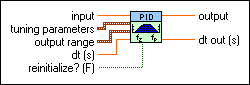input specifies the input value.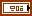tuning parameters specifies the tuning parameters.gain specifies the DC gain. The default is 1. Setting gain to a negative value produces an inverting amplifier with an additional 180-degree phase shift.lag time specifies the phase lag in minutes. A value of zero turns off the lag.lead time specifies the phase lead in minutes. A value of zero turns off the lead. Large lead time values might result in a wild oscillation of the output.output range specifies the range to which to coerce the control output. The default range is –100 to 100, which corresponds to values specified in terms of percentage of full scale. You can change this range to something that is appropriate for your control system. For example, you can relate engineering units to engineering units instead of percentage to percentage. This VI implements integrator anti-windup when the controller output is saturated at the specified minimum or maximum values.output high specifies the maximum value of the controller output. The default is 100.output low specifies the minimum value of the controller output. The default is –100.dt (s) specifies the loop-cycle time, or interval in seconds, at which this VI is called. If dt (s) is less than or equal to zero, this VI calculates the time since it was last called using an internal timer with 1 ms resolution. If dt (s) must be less than 1 ms, specify the value explicitly. The default is -1.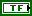reinitialize? specifies whether to reinitialize the output to the current input value.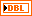output returns the control output of the PID algorithm that is applied to the controlled process.dt out (s) returns the actual time interval in seconds. dt out (s) returns either the value of dt (s) or the computed interval if you set dt (s) to –1.

## PID Lead-Lag (DBL Array)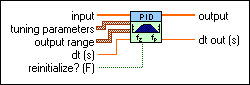input specifies the input value.tuning parameters specifies the tuning parameters.gain specifies the DC gain. The default is 1. Setting gain to a negative value produces an inverting amplifier with an additional 180-degree phase shift.lag time specifies the phase lag in minutes. A value of zero turns off the lag.lead time specifies the phase lead in minutes. A value of zero turns off the lead. Large lead time values might result in a wild oscillation of the output.output range specifies the range to which to coerce the control output. The default range is –100 to 100, which corresponds to values specified in terms of percentage of full scale. You can change this range to something that is appropriate for your control system. For example, you can relate engineering units to engineering units instead of percentage to percentage. This VI implements integrator anti-windup when the controller output is saturated at the specified minimum or maximum values.output high specifies the maximum value of the controller output. The default is 100.output low specifies the minimum value of the controller output. The default is –100.dt (s) specifies the loop-cycle time, or interval in seconds, at which this VI is called. If dt (s) is less than or equal to zero, this VI calculates the time since it was last called using an internal timer with 1 ms resolution. If dt (s) must be less than 1 ms, specify the value explicitly. The default is -1.reinitialize? specifies whether to reinitialize the output to the current input value.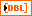output  returns the control output of the PID algorithm that is applied to the controlled process. This VI determines the length of the output from the size of the input array.dt out (s) returns the actual time interval in seconds. dt out (s) returns either the value of dt (s) or the computed interval if you set dt (s) to –1.

## PID Lead-Lag Details

You can use the DBL Array instance of this polymorphic VI in multi-loop PID control applications. In this case, the length of input determines the length of the output array. Other input arrays do not necessarily need to be the same length as input. This VI resizes other input arrays to the same length as input as follows:

• If the input array is longer than input, the input array is truncated to the length of input. Additional values in the array are not used.
• If the input array is shorter than input, the last value of the input array is repeated until the size matches that of input.

In this manner, an input value that must be used for each output calculation does not need to be specified repeatedly in the array passed into this VI. Instead, the array can consist of a single value that is used for each output calculation.

## Example

Refer to the Simulation - Cascade and Feedforward Surge Tank Level VI in the labview\examples\control\PID directory for an example of using the PID Lead-Lag VI.